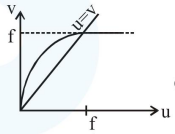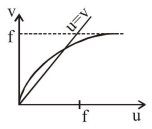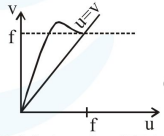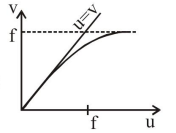# For a concave lens of focal length

Question:

For a concave lens of focal length $\mathrm{f}$, the relation between object and image distance $u$ and $v$, respectively, from its pole can best be represented by ( $u=v$ is the reference line):

1.2.3.4.Correct Option: 4,

Solution:

$v=\frac{u f}{u+f}$

Case-I

If $\quad \mathrm{v}=\mathrm{u}$

$\Rightarrow \mathrm{f}+\mathrm{u}=\mathrm{f}$

$\Rightarrow \mathrm{u}=0$

Case-II

If $u=\infty$

then $v=f$

Only option (4) satisfies this condition.